# 小小秘笈㈠㊦：无编码词条自动造词的小小不足

——看Excel和TextPro如何实现码表精准造词

• 首次码与首尾码
• 等长码与非等长码

ce2=p11+p12+p21+p22

code_e2=p11+p1-1+p21+p2-1
code_e3=p11+p21+p31+p3-1
code_a4=p11+p21+p31+n1-1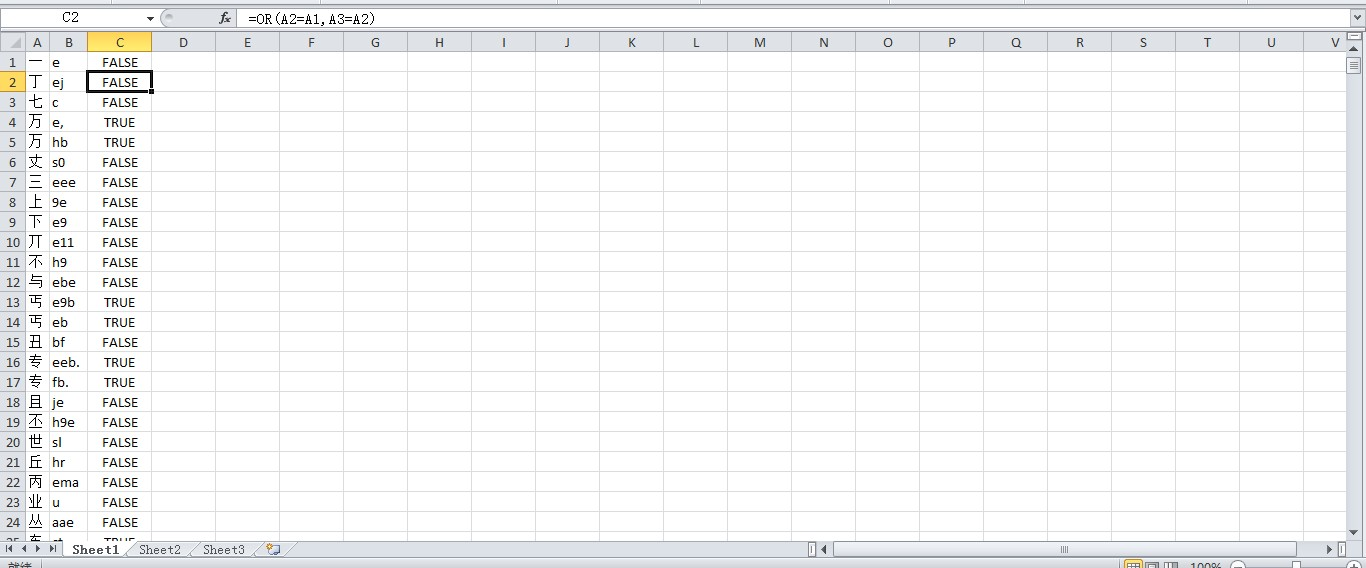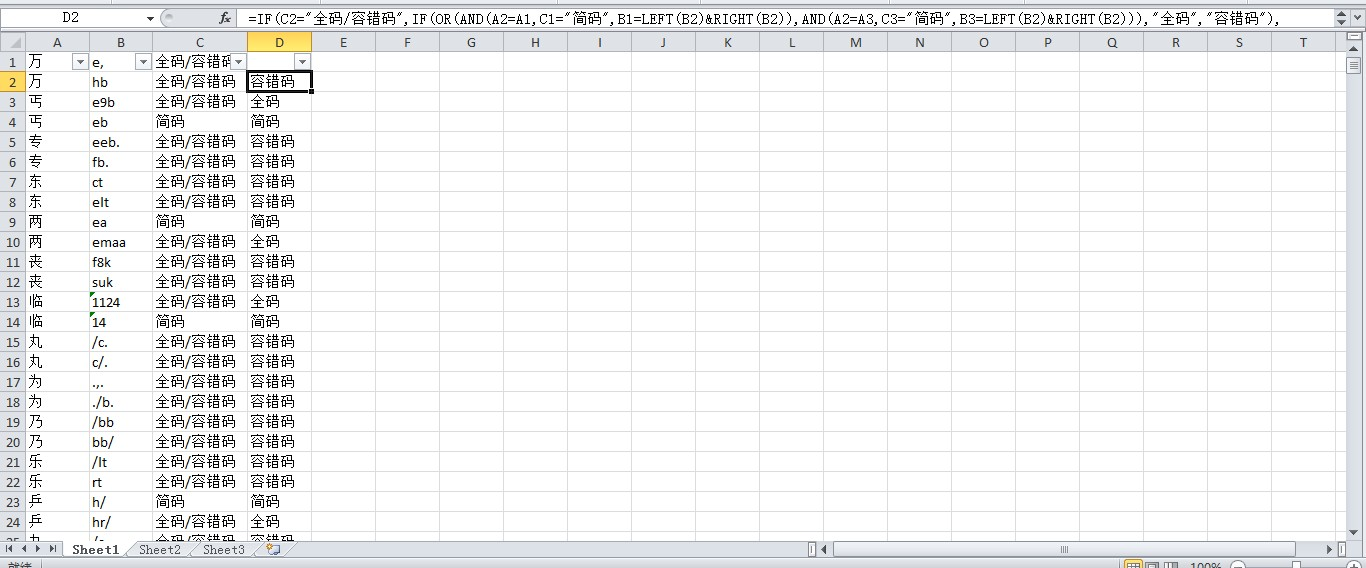C2=IF(LEN(B1)=2,IF(OR(B2=LEFT(B3)&RIGHT(B3),B2=LEFT(B1)&RIGHT(B1)),"简码","全码/容错码"),"全码/容错码")
D2=IF(C2="全码/容错码",IF(OR(AND(A2=A1,C1="简码",B1=LEFT(B2)&RIGHT(B2)),AND(A2=A3,C3="简码",B3=LEFT(B2)&RIGHT(B2))),"全码","容错码"),"简码")

=VLOOKUP(LEFT(E2),\$B\$2:\$D\$7382,3,)&VLOOKUP(RIGHT(E2),\$B\$2:\$D\$7382,3,)

=IF(LEN(F2)<4,F2,"")

=VLOOKUP(LEFT(H2),\$B\$2:\$D\$7382,2,)&VLOOKUP(MID(H2,2,1),\$B\$2:\$D\$7382,2,)&VLOOKUP(RIGHT(H2),\$B\$2:\$D\$7382,3,)

=IF(LEN(I2)<4,I2,"")

• 要查找的值：LEFT(E2)和RIGHT(E2)，即二字词内容（本例中LEFT(E2)值为单字“爱”，RIGHT(E2)值为单字“戴”）。
• 要在其中查找值的区域：\$B\$2:\$D\$7382，锁定单字、首码和构词码共三列的区域（如果写成B:D则是逐行查找而非锁定区域从顶到底查找），其中要查找的值要求位于区域最左列。
• 区域中包含返回值的列号：2，即C列的首码（仅用于三字词前两字编码）；3，即D列的构词码（分别用于二字词全部编码和三字词末字编码）。
• 近似匹配或精确匹配：TRUE或FALSE，也可写成1或0。默认值为TRUE或1，即近似匹配。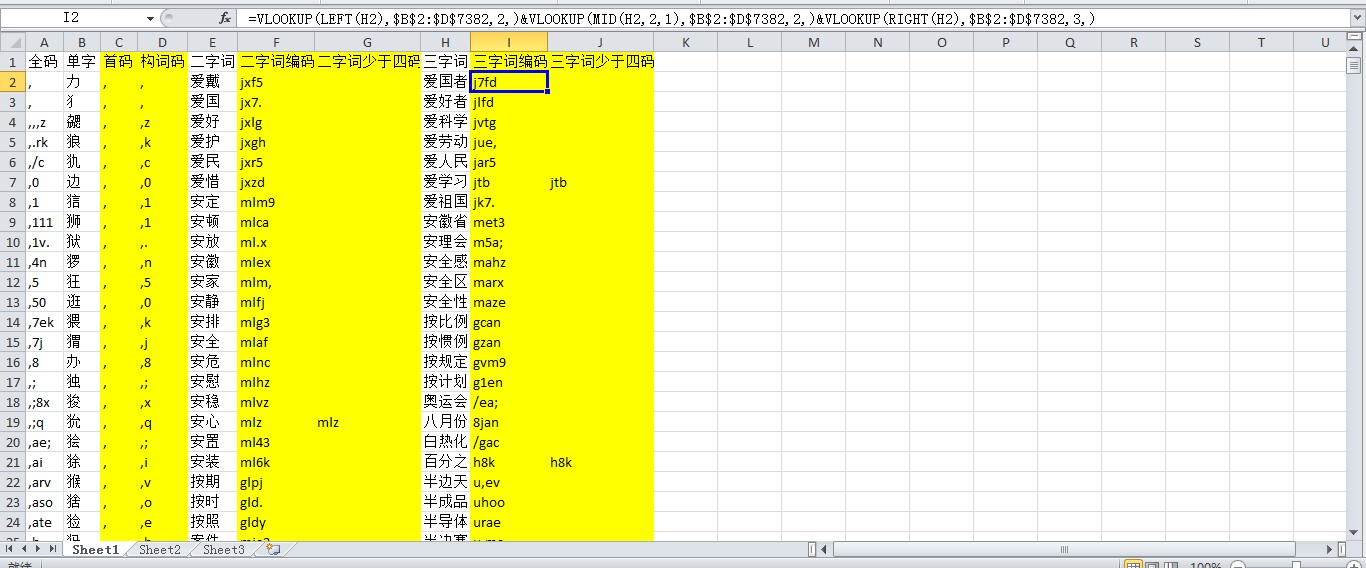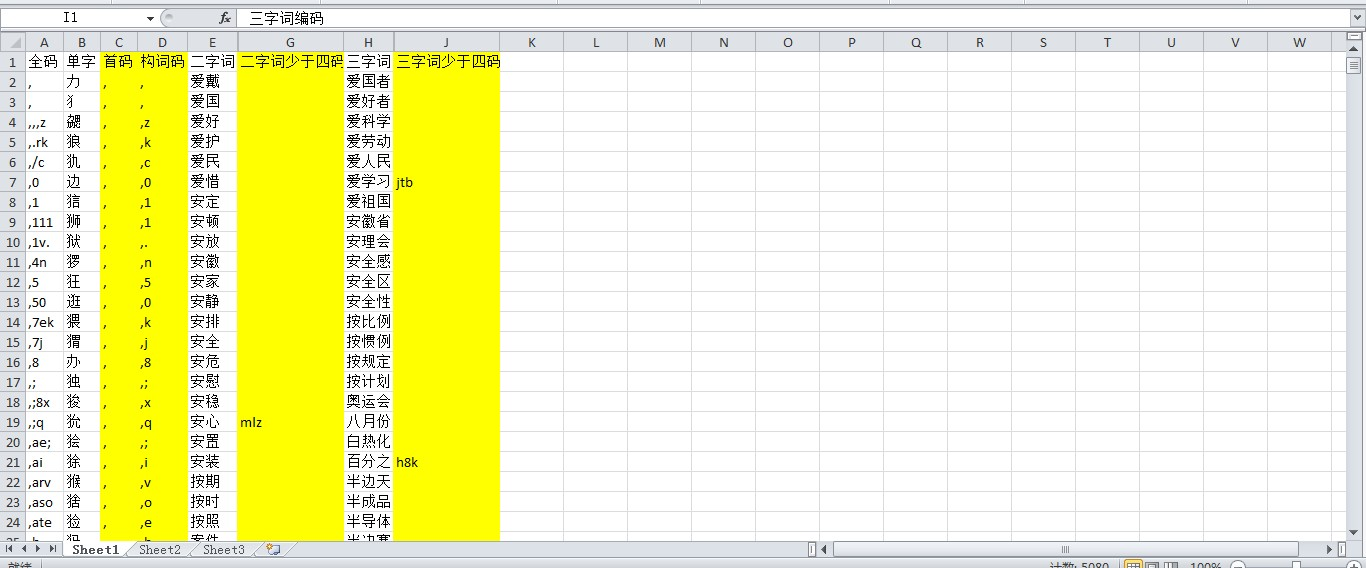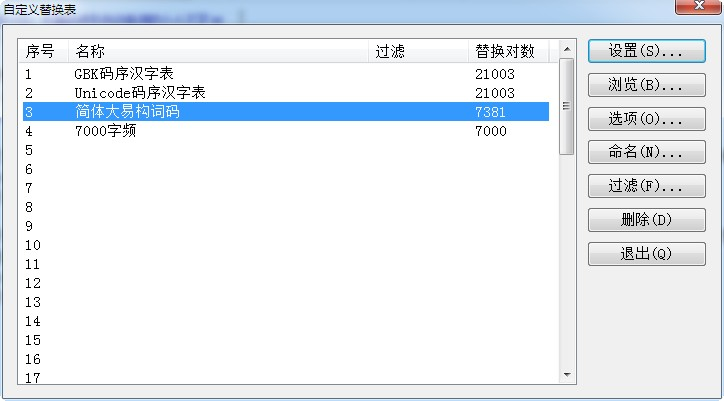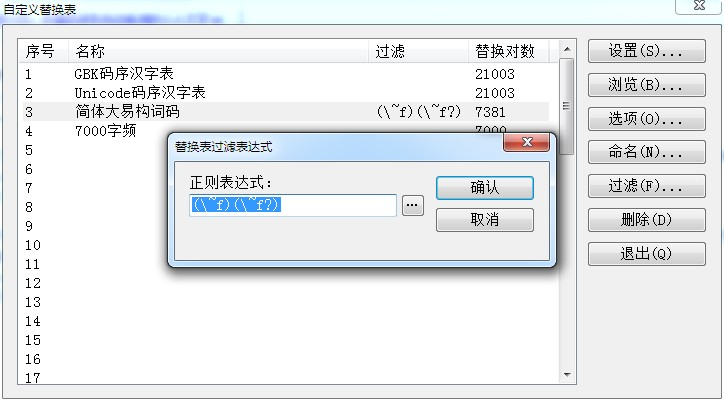新手上路沙发# 发布于：2019-10-18 20:41 分析与建议 为应付台湾输入法造词要求首尾码构词而非首次码构词，传统输入法生成器勉强可以利用构词码解决，小小输入法平台则可以直截了当指定首尾码构词，显然略胜一筹。然而在面对非等长码构词的要求时，不管是传统输入法生成器还是小小输入法平台，两者都力有不逮。在码表预处理时，简体大易码表不像五笔码表可以依靠码长的自然排序来分辨全码、简码和容错码（因为简码与全码总是上下挨着的），而纯粹只能依靠简码来区分全码和容错码。当三者一起出现而且简码与全码之间横亘着容错码时，将导致全码和容错码弄混甚至全部被误判为容错码。看来，第一次粗分简码和全码/容错码的公式不够严谨，有待改进。我们在输出Excel和TextPro二三字词条时还要对满四码的过滤掉其编码，只保留那些少于四码的编码，固然一方面是有意突出小小输入法平台可以自动编码的功能，另一方面则是着眼于一旦单字编码有改动时无需再次为词条重新编码，始终保证词条编码准确有效，同时也减少词条文件的大小。其实小小输入法平台只需稍微改进构词规则算法，引进正则表达式的可有可无的重复指示符即问号（?），那么适用于简体大易的二三字词构词规则可以改写如下：code_e2=p11+p1-1?+p21+p2-1? code_e3=p11+p21+p31+p3-1?就不用大费周章地展开上述两套非等长构词码的解决方案了。 无论如何，为方便用户早日用上小小输入法平台的无编码词条自动造词的功能，在排除码表多码字的过程中无意中发现了通过Excel公式可以对码表中多码字的来源到底是全码、简码或容错码加以识别，算是一项意外收获。唯我本人并非Excel专家，在探索过程中都是先射箭后画靶，才鼓捣出分别适用于两岸较具有代表性的形码输入法的码表多码字自动识别公式。由于五笔和大易的取码规则都大相径庭，反映在码表多码字自动识别公式当然也就大异其趣，往后也许可以成为分析形码输入法码表结构的特征之一。
 新手上路板凳# 发布于：2019-11-01 16:18 经过提炼后，简体大易码表多码字溯源公式已完美实现辨识简码、全码和容错码 关键就是先找出B列码长列于C列，然后按A列和C列进行两级排序（见附图），这样就可强制规定多码字的简码位于全码或容错码之上。同时在辨识全码和容错码时，还需分别输入E2项和E3项，从E3项开始往下自动生成公式结果。值得一提的是E列偶尔会出双全码，那是正常的，因为不管全码还是容错码，其首尾码都与简码完全匹配。这只好留待人来做出判断，正如那些没有简码的多码字无从判断其为全码或容错码。 有关公式如下： D1=IF(LEN(B1)=2,IF(OR(AND(A1=A2,B1=LEFT(B2)&RIGHT(B2)),AND(A1=A3,B1=LEFT(B3)&RIGHT(B3))),"简码","全码/容错码"),"全码/容错码") E2=IF(D2="全码/容错码",IF(AND(A2=A1,D1="简码",B1=LEFT(B2)&RIGHT(B2)),"全码","容错码"),"简码") E3=IF(D3="全码/容错码",IF(OR(AND(A3=A2,D2="简码",B2=LEFT(B3)&RIGHT(B3)),AND(A3=A1,D1="简码",B1=LEFT(B3)&RIGHT(B3))),"全码","容错码"),"简码")
 新手上路地板# 发布于：2019-11-01 16:18 附图：
 新手上路4楼# 发布于：2020-01-14 10:29 通过学习楼主的文章我对小小有了进一步的理解，很多年前使用小小的时候只是把别人整理好的文件拿过来使用，由于我本来并不是一个对计算机精通的人员，所以无法整理成自己想要的词库，往往是哪个平台上面的词库较好，我就转到哪个平台，特别是后来从五笔86转到五笔091后，更是如此。看了楼主的文章，即使没有完全使用楼主的方法，也相当于给我打了好大的一扇窗。想问一下楼主，正则表达式在哪里可以学习？谢谢！期待楼主的进一步更新。
 新手上路5楼# 发布于：2020-01-14 16:46 wnlei：通过学习楼主的文章我对小小有了进一步的理解，很多年前使用小小的时候只是把别人整理好的文件拿过来使用，由于我本来并不是一个对计算机精通的人员，所以无法整理成自己想要的词库，往往是哪个平台上面的词库较好，我就转到哪个平台，特别是后来从五笔86转...回到原帖 小小秘笈㈠㊤的目标正是要解决随意换词库的需要，目前无编码词条自动造词的功能似乎仅见于小小平台。不管《GB/T 15732—1995汉字键盘输入用通用词语集》还是《现代汉语常用词表（草案）》，它们都具有官方背景，其规范词库无疑略胜于民间收集的词库。 至于如何学习正则表达式，建议下载佛典的TextPro，皆因其正则表达式经过大量增改，增强了其对中文的支持。我是原原本本从帮助说明学起的。 下一次秘笈是关于小小平台的本地文本查询，即字典功能。您需要登录后才可以回帖，登录 或者 注册